QlikView App Dev

Discussion Board for collaboration related to QlikView App Development.

Announcements
QlikWorld 2022, LIVE in Denver CO., May 16-19, 2022. REGISTER NOW TO RECEIVE EARLY BIRD PRICING
cancel
Showing results for
Did you mean: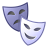Contributor III

Handle Null Dimension in Pivot Table

Hi All,

I got a pivot table as below where product #bbb contain null time bucket.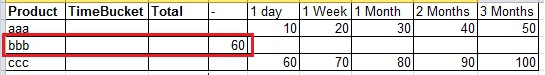I got request to suppress the value of null time bucket for product #bbb by manual assign (60 * 40%) into 1 Week & (60 * 60%) into 2 Months time bucket as below.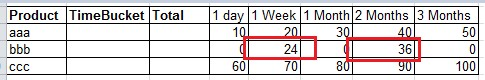Appreciate if anyone can help to provide solution.

Thanks,

Boon Haw

9 RepliesMVP

May be like this:

If(Product = 'bbb', if(TimeBucket = '1 week', Sum(TOTAL <Product> Measure) * 0.40, If(TimeBucket = '2 months', Sum(TOTAL <Product> Measure) * 0.60)), Sum(Measure))MVP

Here is a sample for you to look at:Contributor III
Author

Hi Sunny,

Thanks a lot for your effort.

From the expression below, the product is set as #bbb, in case there are a lot of product and have no idea which product has null time bucket. How can we find out which product has null time bucket.

If(Product = 'bbb', if(TimeBucket = '1 Week', Sum(TOTAL <Product> Sales) * 0.40, If(TimeBucket = '2 Months', Sum(TOTAL <Product> Sales) * 0.60, 0)), Sum(Sales))MVP

You can try this:

If(Sum({<TimeBucket = {'*'}>}Value) > 0, Sum(Value),

If(TimeBucket = '1 Week', Sum(TOTAL <Product> Value) * 0.40,

If(TimeBucket = '2 Months', Sum(TOTAL <Product> Value) * 0.60, 0)))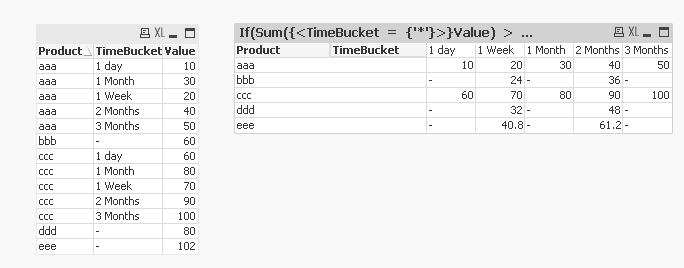Contributor III
Author

Hi Sunny, Thanks a lot for your effortMVP

No problem at all. Does this give you what you were looking for? If it did, would you be able to close this thread by marking correct and any helpful responses?

Qlik Community Tip: Marking Replies as Correct or Helpful

Best,

SunnyContributor III
Author

Hi Sunny,

Recently the user request to show partial sums in pivot.

But unfortunately the total value show "-".

Would need your help to check if can show the partial sums value for product bbb.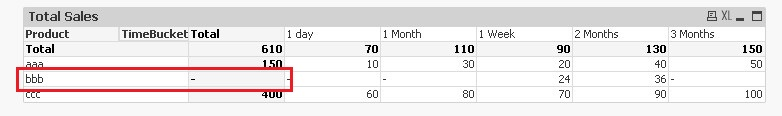MVP

Try this:

If(Product = 'bbb' and SecondaryDimensionality() = 1 and Dimensionality() = 1,

If(TimeBucket = '1 Week', Sum(TOTAL <Product> Value) * 0.40,

If(TimeBucket = '2 Months', Sum(TOTAL <Product> Value) * 0.60, 0)), Sum(Value))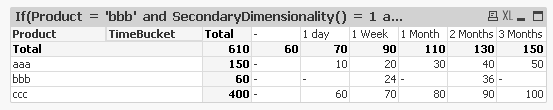Contributor III
Author

Thanks a lot Sunny, the solution work perfectly.Community Browser